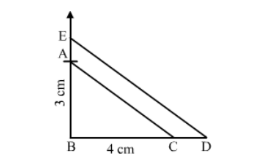# Draw ∆ABC, right-angled at B, such that AB = 3 cm and BC = 4 cm.`
Question:

Draw $\triangle A B C$, right-angled at $B$, such that $A B=3 \mathrm{~cm}$ and $B C=4 \mathrm{~cm}$. Now, construct a triangle similar to $\triangle A B C$, each of whose sides is $\frac{7}{5}$ times the corresponding sides of

ABC.

Solution:

Step 1. Draw a line segment BC = 4 cm.
Step 2. With B as centre, draw an angle of 90o.
Step 3. With B as centre and radius equal to 3 cm, cut an arc at the right angle and name it A.
Step 4. Join AB and AC.
Thus, △ ABC is obtained .

Step 5. Extend BC to D, such that BD $=\frac{7}{5} \mathrm{BC}=\frac{7}{5}(4) \mathrm{cm}=5.6 \mathrm{~cm}$.

Step 6. Draw DE ∥ CA, cutting AB produced to E.Thus, $\triangle \mathrm{EBD}$ is the required triangle, each of whose sides is $\frac{7}{5}$ the corresponding sides of $\triangle A B C$.